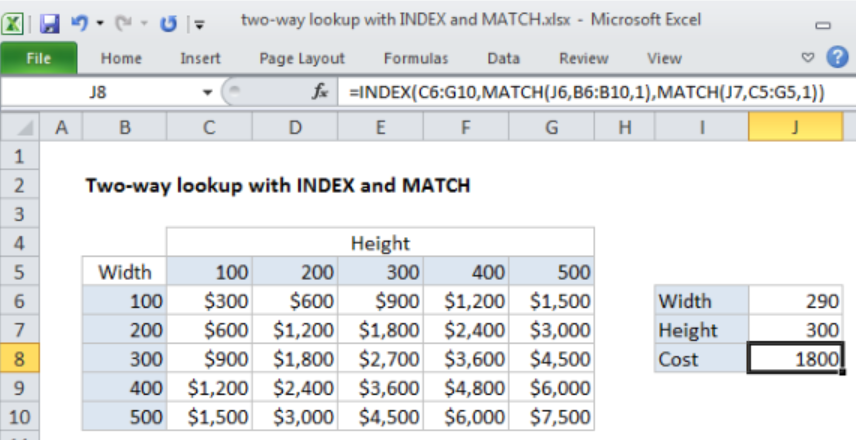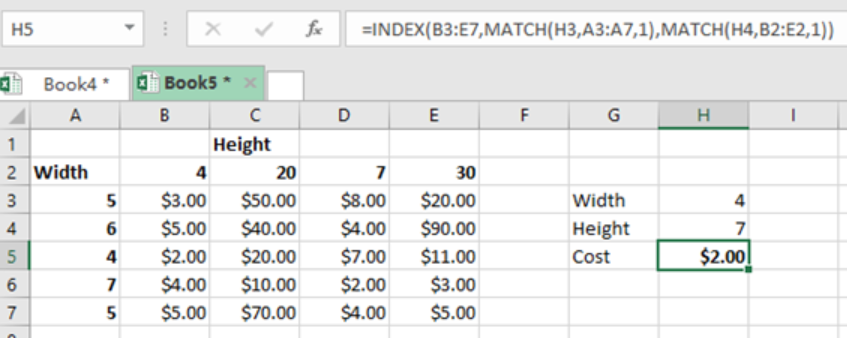Get instant live expert help with Excel or Google Sheets“My Excelchat expert helped me in less than 20 minutes, saving me what would have been 5 hours of work!”

#### Post your problem and you’ll get Expert help in seconds.

Your message must be at least 40 characters
Our professional Expert are available now. Your privacy is guaranteed.

# Two-way lookup with INDEX and MATCH

There are times when we want to lookup a value in an excel table using both the rows and columns. To do this, we need to build a formula that is based on a two-way lookup with INDEX and MATCH as explained in this article.Figure 1: How to do two-way lookup with INDEX and MATCH

## General syntax of the formula

`=INDEX(data, MATCH(val,rows,1),MATCH(val,columns,1))`

## How the formula works

• This formula carries out approximate match. This means that you need to first sort the data before you apply it.
• The INDEX function forms the backbone of the formula. This function retrieves the value from the data as supplied in range C6:G10. This is based on a row number as well as column number.
• This is in the following form; =INDEX(C6:G10, row, column)
• The MATCH function then comes in to help get the row and column number. This function is also configured to approximate match by setting the third argument to 1, implying TRUE.

## ExampleFigure 2:  Example of how to do two-way lookup with INDEX and MATCH

In the above example, we want to find the cost of at the point where the height 7 and width 4 meet. To do this, we proceed as follows;

Step 1: Tabulate the excel table with data

Step 2: Indicate where you want the data to be located, i.e. in column H.

Step 3: Indicate the parameters, i.e. the width (4) and the height (7).

Step 4: Then in the cell where we want to have the cost, we put the formula `=INDEX(B3:E7,MATCH(H3,A3:A7,1),MATCH(H4,B2:E2,1))`

Step 5: We press Enter to get the cost as \$2.00

## Instant Connection to an Expert through our Excelchat Service

Most of the time, the problem you will need to solve will be more complex than a simple application of a formula or function. If you want to save hours of research and frustration, try our live Excelchat service! Our Excel Experts are available 24/7 to answer any Excel question you may have. We guarantee a connection within 30 seconds and a customized solution within 20 minutes.

### Did this post not answer your question? Get a solution from connecting with the expert.Another blog reader asked this question today on Excelchat:
Solution examplesindex and match with duplicates. I need to use another column as a reference, so my return value has two match the value of two things for it to return
Solved by E. H. in 60 minsI need a formula to compare the data in two columns and then export the mismatched data in the 3rd column
Solved by S. Q. in 20 minsHello, I have a big spreadsheet in which I need to know how many patients came in each month based on provider. I am using the following formula but I still get the N/A error. =INDEX('No Show Appts Data'!D:E, MATCH(1, 'No Show Appts Data'!D:D='No Shows Data'!M2)*('No Shows Data'!E:E='No Shows Data'!N1),0)) No Show Appts Data is the name of the sheet where Column D is Month Year of date in question and Column E is the provider. No Shows Data is the sheet where I am making all the formula calculation where Column M is Month and Year and Column N is the provider in question and therefore M2 is the month in question and N1 the provider in question. How do I fix this error? Thanks
Solved by F. H. in 40 minsI need to find an INDEX function that will convert the Call Day (a number 1-7) to the actual weekday found in row 1 of the DayofWeek named range. I don't know if I'm supposed to use the MATCH function as well or not.
Solved by D. D. in 11 minsI have two columns with names and a third one with email addresses. For every name that matches, I need to copy the email address to an empty column next to the matching name. .
Solved by E. U. in 15 mins## Subscribe to Excelchat.coAnother blog reader asked this question today on Excelchat: